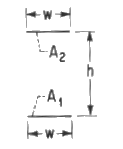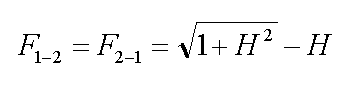Cody

# Problem 2805. Radiation Heat Transfer — View Factors (1)

View factors (aka configuration factors) are utilized in some radiation heat transfer models to estimate heat transfer rates between surfaces. In particular, the thermal energy leaving a given surface is applied to other surfaces, as appropriate, based on how much the hot surface "sees" the other surfaces. As such, view factors are purely geometrical in nature. A range of view factor formulae are available here.

For this problem, calculate the view factor from surface 1 to surface 2 (F_1-2) for two directly opposed, infinitely long plates of the same finite width given the height, which may be a vector of values:### Solution Stats

56.96% Correct | 43.04% Incorrect
Last Solution submitted on May 06, 2020